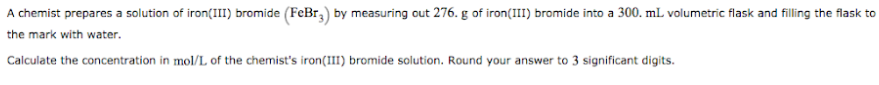# Problem: A chemist prepares a solution of iron(III) bromide (FeBr3) by measuring out 276. g iron(III) bromide into a 300. mL volumetric flask and filling the flask to the mark with water. Calculate the concentration in mol/L of the chemist's iron(III) bromide solution. Round your answer to 3 significant digits.

###### FREE Expert Solution
92% (314 ratings)###### Problem Details

A chemist prepares a solution of iron(III) bromide (FeBr3) by measuring out 276. g iron(III) bromide into a 300. mL volumetric flask and filling the flask to the mark with water. Calculate the concentration in mol/L of the chemist's iron(III) bromide solution. Round your answer to 3 significant digits.